# Fluids, Gases & Heat Flashcards Preview

## AP Physics > Fluids, Gases & Heat > Flashcards

Flashcards in Fluids, Gases & Heat Deck (133):
1

2

3

4

5

6

7

8

9

## How is the buoyancy force of a submerged object calculated?### The buoyancy force of a submerged object is simply the weight of the liquid displaced by the portion of the object that is submerged. FB = ρliq * Vsub* g Where: FB = the buoyancy force pushing up on the object ρliq = the density of the liquid Vsub = the volume of the object that is submerged in the liquid g = the gravitational acceleration (commonly 10 m/s2)10

11

12

13

## A cube of styrofoam, with a density of 0.5 g/cm3, is dropped into water. How much of the cube is submerged in the water when the cube begins to float?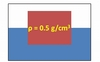### One half of the cube will be submerged in the water. The proportion of an object that is under the surface of a liquid when the object floats is simply equal to ρobj/ρliq, where ρobj is the object's density and ρliq is the liquid's density. In this case, the object is one-half the density of the liquid, and thus one-half of it will be submerged when it floats. Note that if ρobj > ρliq, this fraction is greater than 1, and the object sinks to the bottom.14

15

16

17

18

## What does Pascal's Law predict about the pressure on plates 1 and 2 in the diagram below?### Pascal's Law predicts that the pressures will be equal. The force F1 will be exerted on the fluid behind plate 1 as a pressure P1. The fluid will exert that pressure, undiminished, on all the walls it is contacting, including plate 2.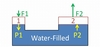19

## How does the force F2 change if plate 2 in the hydraulic lift pictured below doubles in area?### The force F2 doubles. According to Pascal's Law, the pressures on plates 1 and 2 must be equivalent. From the definition of pressure, therefore: P1 = F1/A1 = F2/A2 = P2 So force and area are directly proportional, and doubling A2 will double F2 as well.20

## How does the distance D2 compare to the distance D1 if plate 2 in the hydraulic lift pictured is double the area of plate 1?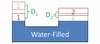### D2 = ½D1. Since the liquid between the plates in incompressible, the volume displaced by plate 1 must be equal to the volume displaced by plate 2: V1 = A1D1 = A2D2 = V2 So distance and area are inversely proportional, and a plate of double the area will move half the distance.21

22

23

24

25

26

27

28

## How does the speed of a fluid flowing through a pipe change, as the diameter of the pipe decreases?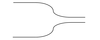### As pipe diameter decreases, fluid flowing through the pipe begins to flow faster. This is akin to placing your thumb partially over the end of a water hose, which causes the water to flow through faster, creating a jet of water.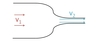29

30

31

32

33

34

35

36

37

38

39

40

## How much does the length of an object change when its temperature changes?

### An object's length change depends on its coefficient of thermal expansion: ΔL/L =αL ΔT Where: L = the object's original length ΔL = the change in the object's length αL = the object's coefficient of thermal expansion ΔT = the change in temperature41

42

43

44

45

46

47

48

49

50

51

52

53

54

55

56

57

58

59

60

61

62

63

64

65

66

67

68

69

70

71

## a material's standard enthalpy of formation, ΔHof

### The standard enthalpy of formation (ΔHof) is the enthalpy change of the formation reaction for the material from its fundamental elements under standard conditions. Ex: The enthalpy of formation for NaCl is  -411.12 kJ mol−1 and follows from the general equation:72

73

74

75

76

77

78

79

80

81

82

83

84

85

86

87

88

## The curve below represents a sample's temperature vs. heat added. What phases (solid, liquid, and/or gas) are present at each labeled point on the plot?89

## The curve below represents a sample's temperature vs. heat added. What heat (q) formula would need to be applied, in order to calculate heat added to the system at each labeled point on the plot?90

91

92

93

94

95

96

97

98

99

100

101

102

103

104

105

106

107

108

109

110

111

112

113

114

115

116

117

118

119

120

121

122

123

124

125

126

127

128

129

130

131

132

133